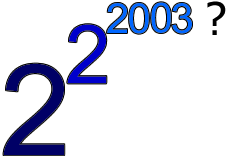#### You may also like### Thirty Six Exactly

The number 12 = 2^2 × 3 has 6 factors. What is the smallest natural number with exactly 36 factors?### Even So

Find some triples of whole numbers a, b and c such that a^2 + b^2 + c^2 is a multiple of 4. Is it necessarily the case that a, b and c must all be even? If so, can you explain why?### Power Crazy

What can you say about the values of n that make $7^n + 3^n$ a multiple of 10? Are there other pairs of integers between 1 and 10 which have similar properties?

# Lastly - Well

##### Age 11 to 14Challenge LevelDid you spot that, without brackets, this could be either 2 to the power $2^{2003}$ or 4 to the power 2003? The solutions below took the first interpretation.

A group of students from the Mount School in York and Andrei of School 205, Bucharest sent in solutions. Well done to all of you. This problem relied on you identifying the cycle in the last two digits of the solution.

Below is a table which shows the last two digits for each of the powers in a full cycle from 04 back to 04:

$$\begin{array}{c} 2^1 \rightarrow 02\\ 2^2 \rightarrow 04\\ 2^3 \rightarrow 08\\ 2^4 \rightarrow 16\\ 2^5 \rightarrow 32\\ 2^6 \rightarrow 64\\ 2^7 \rightarrow 28\\ 2^8 \rightarrow 56\\ 2^9 \rightarrow 12\\ 2^{10} \rightarrow 24\\ 2^{11} \rightarrow 48\\ 2^{12} \rightarrow 96\\ 2^{13} \rightarrow 92\\ 2^{14} \rightarrow 84\\ 2^{15} \rightarrow 68\\ 2^{16} \rightarrow 36\\ 2^{17} \rightarrow 72\\ 2^{18} \rightarrow 44\\ 2^{19} \rightarrow 88\\ 2^{20} \rightarrow 76\\ 2^{21} \rightarrow 52 \\ 2^{22} \rightarrow 04 \end{array}$$

From the table you can see that the last two digits of powers of 2 go in cycles of 20 and so $2^{2003}$ will end in the two digits 08. We only need to look at the last two digits in the final stage of the solution because we are always looking at cycles of 20 - one digit is not enough (it would only enable us to cover all possibilities in a cycle of ten or less) and three digits is more than we need.

But we need to raise 2 to the power $2^{2003}$ and identify what happens to the last digits in this case.

As the pattern of the last two digits will also be based on the same cycle we can use the table to see what the last two digits will be for a power ending 08 (which is 8 mod 20) and this is 56.